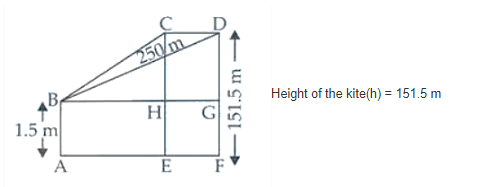# A kite is moving horizontally at a height`
Question:

A kite is moving horizontally at a height of $151.5$ meters. If the speed of kite is

10 m/s, how fast is the string being let out; when the kite is 250 m away from

the boy who is flying the kite? The height of boy is 1.5 m.

Solution:Speed of the kite(V) = 10 m/s

Let FD be the height of the kite and AB be the height of the kite and AB be the height of the boy.

Now, let AF = x m

So, BG = AF = x

And, dx/dt = 10 m/s

From the figure, it’s seen that

GD = DF – GF = DF – AB

= (151.5 – 1.5) m = 150 m [As AB = GF]

Now, in ∆ BDG

BG2 + GD2 = BD2 (By Pythagoras Theorem)

x2 + (150)2 = (250)2

x2 + 22500 = 62500

x2 = 62500 – 22500 = 40000

x = 200 m

Let initially the length of the string be y m

So, in ∆ BDG

BG2 + GD2 = BD2

x2 + (150)2 = y2

Differentiating both sides w.r.t., t, we have

$2 x \cdot \frac{d x}{d t}+0=2 y \cdot \frac{d y}{d t} \quad\left[\because \frac{d x}{d t}=10 \mathrm{~m} / \mathrm{s}\right]$

$2 \times 200 \times 10=2 \times 250 \times \frac{d y}{d t}$

$\frac{d y}{d t}=\frac{2 \times 200 \times 10}{2 \times 250}=8 \mathrm{~m} / \mathrm{s}$

Therefore, the rate of change of the length of the string is 8 m/s.